7. THE LAW OF THE RADIATION OF THE PERFECT BLACKBODY IS

THE LAW OF CLASSICAL  PHYSICS

7.1. General

Hitherto our search has been conducted within the framework of the laws of classical physics. But it is known that at the end of the 19th century it was announced that the law of classical physics operate successfully only in the macro world, and in the microworld the different, quantum physical laws operate. This point of view predominated during the whole 20th century. Now when we have determined the models of the photon and the electron on the basis of the laws of classical physics, a question arises: aren’t the physicists of the former generations mistaken when they have burried the possibility of classical physics to solve the problems of in the microworld? In order to give a reply to this question, let us analyse attentively the sources of misbelief to classical physics to solve the problems of  the microworld. Everything has begun from the deduction of the law of radiation of the perfect blackbody.

The deduction of mathematical model of the distribution law of the perfect blackbody made by Max Planck at the beginning of the 20th century was based on notions and ideas, which are considered to contradict the laws of classical physics , . Planck introduced constant h with dimensionality of mechanical action into the mathematical model of distribution law of the perfect blackbody; it contradicted to the electromagnetic nature of radiation. Nevertheless, his mathematical model described experimental dependencies of this radiation. The constant introduced by him pointed out to the fact that radiation takes place not continuously, but in packets. It contradicted to Reyleigh - Jeans law, which was based on classical notions concerning wave nature of electromagnetic radiation, but described experimental dependencies in the low frequency range only .

As the mathematical model of Reyleigh - Jeans law is present in the mathematical model of the distribution law of the perfect blackbody, it means that Planck distribution law of the perfect blackbody is based on the mutually exclusive wave and corpuscular notions on nature of radiation , .

Continuous wave process of radiation with portal process was a safe basis for acknowledgement of classical physics. Since that time the physics began to think that the application field of the laws of classical physics is restricted by the macro world. They think that other, quantum laws operate in the micro world, that’s why physics, which describes the microworld, should be called quantum physics. It should be noted that Max Planck tried to understand the mixture of such physical notions and return them to the classical way of development, but failed , , . For the first time, the mathematical model of the law of the radiation of the perfect blackbody connected with thermodynamic ideas was received by Yu.M. Ageev , .

Years later, we have to admit that the border between the law of classical physics and quantum physics has not been established yet. Still it is difficult to solve many problems of the macro world, and many problems are considered to be unsolved within the framework of the existing notions and conceptions, that’s why we have to return to an attempt of Max Planck to derive a mathematical model of the law of the perfect blackbody on the basis of classical notions , .

# 7.2. Theoretical  Part

First of all, let us give  Reyleigh - Jeans formula, which describes  satisfactory experimental behaviour of a low frequency range of radiation.  Taking into consideration the wave conceptions on electromagnetic radiation they have found out that energybeing available in volume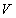of the perfect blackbody is determined by the dependence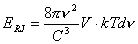,                                                             (185)

where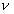is frequency of radiation;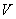is volume of space of the perfect blackbody;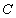is the speed of light;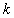is Boltzmann constant;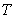is absolute radiation temperature.

If we divide the left part and the right part of the relation (185) by volume, we  get volumetric density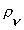of electromagnetic radiation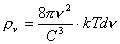.                                                                    (186)

The deduction of this formula is based  the notion  a number of standing waves of electromagnetic radiation with frequencyin the closed space of the perfect blackbody .

In order to get the mathematical model, which could describe the whole range of electromagnetic radiation of the perfect blackbody, Max Planck postulated that the radiation is not  continuous but it is in packets, and  energy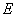of each radiated packet is equal to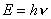, and the formula for the calculation of density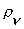of electromagnetic radiation of the perfect blackbody is as follows (Fig. 19)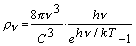.                                                             (187)Fig. 19.  The experimental law of the radiation of black body

Value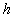is a constant with mechanical dimensionality of the action. The essence of this action was completely unclear at that time. Nevertheless, the mathematical model obtained by Planck gave a satisfactory description of the experimental laws  of the radiation of the  perfect blackbody.

As it is clear, the expression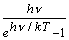in  (187)  significant addition to  Reyleigh - Jeans formula (186),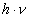energy of one radiated photon.

In order to understand the physical essence of Planck addition, one should have a notion of  electromagnetic structure of the photon, because the physical essence of Planck constant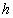is in this structure.  As the product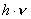describes energies of the photons  the whole scale of electromagnetic radiation,  electromagnetic structure of the photon is concealed in  dimensionality of Planck constant.

We have found out that the photon has  rotating electromagnetic structure, the centre of mass describes the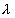, which is equal to radius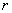of its rotation.  As a result,  of  constant is as follows (88)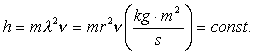(88)

As it is clear, Planck constant has  clear mechanical dimensionality of angular momentum, which has another name –  moment of momentum.  It is known  that the law of conservation of angular momentum governs  constancy of angular momentum, and the reason  clear.  First of all, the notion “the law of conservation of angular momentum” is  notion  classical physics, to be more precise,  classical Newtonian mechanics. It means that if no external force influences a rotating solid body,  angular momentum of such  body remains constant.

The photon is not a solid body but it has mass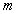, and we have every reason to believe  photon an electromagnetic substance (i.e. electromagnetic field) rotating in relation to the axis plays the role of mass.  It results from the mathematical model (88) of Planck constant that the electromagnetic model of the photon should be such that  simultaneous change of mass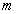, radius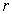and frequency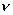of the rotating electromagnetic fields of the photon would retain their product  reflected in the mathematical expression of Planck constant  (88) . The diagram of the rotating electromagnetic fields of the photon is  in Fig. 5.

It is known that if  mass (energy) of  photon is increased, its wavelength is decreased. This change is implemented with the help of Planck constant (88) in the model of the photon (Fig. 5) .

As the law of conservation of angular momentum (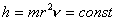) governs  constancy of  constant,  density of their electromagnetic fields is increased when the mass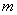of the photon (Fig. 5) is increased, and due to the electromagnetic forces which are balanced by an equal the centrifugal forces of inertia compressing the photon.   This leads to the reduction of radiusof  photon rotation, which is always equal to its wave length.  But as radiusin the expression of  Planck constant is squared,  oscillation frequencyshould  be increased in order to preserve constancy of Planck constant (88).  Due to this fact, a small alteration  mass of the photon changes its rotation radius and frequency automatically in such a way that angular momentum (Planck constant) remains constant.  Thus, the photons of all frequencies preserving their electromagnetic structure change mass, frequency and rotation radius in such a way that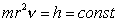It means that the law of conservation of angular momentum governs the principle of this alteration , .

Why the photons of all frequencies move in vacuum with equal speed?  The answer is alteration of frequencyof the photon is a consequence of the alteration of the  mass, which changes  the density of the electromagnetic fields of the photon and the  electromagnetic  and centrifugal forces of inertia  leads to the change  radius of its rotation, which is always equal to  its wavelength.  The above-mentioned changes take place in such a way that the product of frequency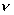and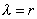constant for the photons of all frequencies and is equal to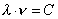.  The speed of the centre of masses M of the photon (Fig. 5) is changed  in such a way that its average value remains constant and equal to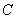(Fig. 7) .

Thus, the law of conservation of angular momentum, one of the fundamental laws of classical physics (to be more precise, classical mechanics) governs  constancy of Planck constant.  In modern physics  in classical mechanics it is called the law of conservation of  moment of momentum .  It is a purely classical mechanic law, not a mystical mechanical action as it has been considered previously.  That is why the appearance of  constant in the mathematical model  of the law  of the radiation of the  perfect blackbody does not give the reason to consider that  classical physics is unable to describe the distribution process of this body.  On the contrary, the law of conservation of angular momentum, the most fundamental law of classical physics, takes part in the description of this process , , .  Thus, Planck  law of the radiation of the perfect blackbody is a law of classical physics.  The derivation of this law is based on classical notions  given below.

# 7.3. Classical  Derivation  of  the  Law  of  the Radiation of  the  Perfect  Blackbody

Use  Reyleigh - Jeans ideas in the calculation of a number of packets of electromagnetic radiation in the space of the perfect blackbody,  describe a separate packet of electromagnetic radiation not as the standing wave, but  as the photon (Fig. 5)  , , , ,  .  As  radii of the closed electrical fields of the photon are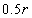and radii of the centre mass of electromagnetic fields arethe photon does not have   a spherical form, but  a flat form  is nearly one fourth of the sphere with  radius.  Thus, the volume of the localized space, in which the photon can be present, will be nearly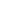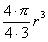.  Volume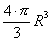of the spherical space of radius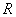of the perfect blackbody will  many orders of magnitude greater than the volume of the photon.  Maximal quantity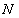of the photons in this space (as well as  maximal quantity of standing waves in  Reyleigh - Jeans formula)  will be determined by a dependence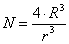.                                                                               (188)

Taking into consideration that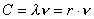, we’ll have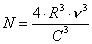.                                                                           (189)

The interval of frequencies fromto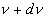, the number  will be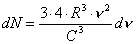.                                                                        (190)

As the photon moves linearly and rotates round its axis in three-dimensional Euclidean space, it will have six grades of freedom.  Taking  into consideration and dividing the left and the right parts (190) by volume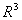,   volumetric density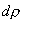of   photons in the spherical space of the perfect blackbody as shown below: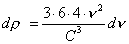.                                                                        (191)

We integrate, we get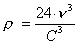(192)

Thus, we have  volumetric density (192) of the photons in the spherical space of the perfect blackbody.  If  spherical space has a small hole,  energy that is emitted via this hole, will depend  energy of each photon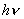, to be more precise, on its frequency. Then the photons, which have passed via  hole in the spherical space, will be absorbed.  As  energy of each photon on the plane of its polarization is realized by two grades of freedom, the value of heat energy of the absorbed photons will be equal to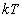.  It  means that volumetric density  of the radiation of the perfect blackbody will depend on energyof each radiated photon and energyof the whole set  photons.

As  the radiation of the perfect blackbody is a set of the photons, each of which has only kinetic energy, we should introduce kinetic energy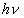of the photon and heat energyof the set of the radiated photons into the mathematical model of the distribution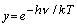.                                                                              (193)

We should take into account that the photons are emitted by the electrons of the atoms during their energy transitions.  Each electron can make a series of transitions  levels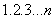emitting the photons of various energy.  The complete distribution of volumetric density of energy  emitted photons will consist of a sum of the distributions, which take into account  energies of the photons of all energy levels.  With due regard for the above - mentioned facts, Maxwell law, which takes into account  energy distribution of the photons  all (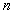) energy levels of the atom,  be put down in the following way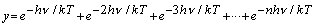,                               (194)

whereis the main quantum number  determines  of the position of electron in the atom.

It is known that sum of row (194) is equal to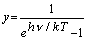.                                                              (195)

If we multiply the right part of volumetric density of the photons (192) in the space of the perfect blackbody by a mathematical expression (195) of the distribution law of this density and by  energy of one photon, we  get  volumetric density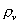of energy  which is radiated  by the perfect blackbody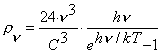.                                                          (196)

It is the  law of the radiation of the  perfect blackbody (187) as obtained by Max Planck.  Expression (196) differs from expression (187)  coefficient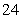.  According to E.V. Shpolsky , the value of this coefficient depends on the character of  electromagnetic radiation and can vary from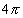to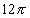.  But within the framework of the above-mentioned notions, the  coefficient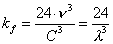(197)

acquires a precise physical sense.  It characterizes (as it should)  density of the photons in the cavity of the perfect blackbody.

The   value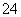does not change the  law of the radiation of the  perfect blackbody, why  more precise value of this  coefficient  can be determined by experiment.

Thus, we have derived the  law of the radiation of the perfect blackbody on the grounds of purely classical notions and ideas, and we see no reason to believe that this law contradicts classical physics.  On the contrary, it follows from  the laws of this physics. . All components of the mathematical model of the law (196) of radiation of the perfect blackbody have got an appropriate clear physical sense.

The Foundations of Physchemistry of Microworld

Ó2003 Kanarev Ph. M.

Internet Version - http://book.physchemistry.innoplaza.net

<< Back to Physchemistry Book Index